# Selina Concise Physics Class 10 ICSE Solutions Work, Energy and Power

## Selina Concise Physics Class 10 ICSE Solutions Work, Energy and Power

APlusTopper.com provides step by step solutions for Selina Concise ICSE Solutions for Class 10 Physics Chapter 2 Work, Energy and Power. You can download the Selina Concise Physics ICSE Solutions for Class 10 with Free PDF download option. Selina Publishers Concise Physics for Class 10 ICSE Solutions all questions are solved and explained by expert teachers as per ICSE board guidelines.

Selina ICSE Solutions for Class 10 Physics Chapter 2 Work, Energy and Power

Exercise 1(A)

Solution 1.

Work is said to be done only when the force applied on a body makes the body move. It is a scalar quantity.

Solution 2.

(i) When force is in direction of displacement, then work done , W = F x S
(ii) When force is at an angle θ to the direction of displacement, then work done, W= F S cos θ

Solution 3.

(a) When force is at an angle θ to the direction of displacement, then work done, W= F S cos θ
(b) (i) For zero work done, the angle between force and displacement should be 90o as cos 90= 0
W = FS cos90o= FS x 0 = 0
(ii) For maximum work done, the angle between force and displacement should be 0o as cos0= 1
Hence, W=FScos 0o=FS

Solution 4.

Two conditions when the work done is zero are:

1. When there is no displacement (S = 0) and,
2. When the displacement is normal to the direction of the force (θ = 90o).

Solution 5.

(i) If the displacement of the body is in the direction of force, then work done is positive.
Hence, W= F x S
For example: A coolie does work on the load when he raises it up against the force of gravity. The force exerted by coolie (=mg) and displacement, both are in upward direction.

(ii) If the displacement of the body is in the direction opposite to the force, then work done is negative.
Hence, W =- F x S
For example: When a body moves on a surface, the frictional forces between the body and the surface is in direction opposite to the motion of the body and so the work done by the force of friction is negative.

Solution 6.

Work is done against the force.

Solution 7.

When a body moves in a circular path, no work is done since the force on the body is directed towards the centre of circular path (the body is acted upon by the centripetal force), while the displacement at all instants is along the tangent to the circular path, i.e., normal to the direction of force.

Solution 8.

Work done by the force of gravity (which provides the centripetal force) is zero as the force of gravity acting on the satellite is normal to the displacement of the satellite.

Solution 9.

Work is done only in case of a boy climbing up a stair case.

Solution 10.

When a coolie carrying some load on his head moves, no work is done by him against the force of gravity because the displacement of load being horizontal, is normal to the direction of force of gravity.

Solution 11.

Force applied by the fielder on the ball is in opposite direction of displacement of ball. So, work done by the fielder on the ball is negative.

Solution 12.

When a coolie carries a load while moving on a ground, the displacement is in the horizontal direction while the force of gravity acts vertically downward. So the work done by the force of gravity is zero.

Solution 13.

S.I unit of work is Joule.
C.G.S unit of work is erg.
Relation between joule and erg :
1joule = 1N x 1m
But 1N = 105dyne
And 1m = 100 cm = 10cm
Hence, 1 joule = 105dyne x 102cm
= 107dyne x cm = 107erg
Thus, 1 Joule = 107 erg

Solution 14.

S. I unit of work is Joule.
1 joule of work is said to be done when a force of 1 newton displaces a body through a distance of 1 metre in its own direction.
Previous

Solution 15.

Relation between joule and erg :
1 joule = 1N x 1m
But 1N = 105dyne
And 1m = 100 cm= 10cm
Hence, 1 joule = 105dyne x 102cm
= 107dyne x cm = 107erg
Thus, 1 Joule = 107 erg

Solution 16.

Let a body of mass m fall down through a vertical height h either directly or through an inclined plane e.g. a hill, slope or staircase. The force of gravity on the body is F = mg acting vertically downwards and the displacement in the direction of force (i.e., vertical) is S=h. Therefore the work done by the force of gravity is
W = FS = mgh

Solution 17.

Let a boy of mass m climb up through a vertical height h either through staircase of using a lift. The force of gravity on the boy is F=mg acting vertically downwards and the displacement in the direction opposite to force (i.e., vertical) is S=-h. Therefore the work done by the force of gravity on the boy is
W= FS =-mgh
or,the work W=mgh is done by the boy against the force of gravity.

Solution 18.

The energy of a body is its capacity to do work. Its S.I unit is Joule (J).

Solution 19.

eV measures the energy of atomic particles.
1eV= 1.6 x 10-19J

Solution 20.

1 J = 0.24 calorie

Solution 21.

Calorie measures heat energy.
1calorie = 4.18 J

Solution 22.

1kWh is the energy spent (or work done) by a source of power 1kW in 1 h.
1kWh = 3.6 x 106J

Solution 23.

The rate of doing work is called power. The S.I. unit of power is watt (W).

Solution 24.

Power spent by a source depends on two factors:
(i) The amount of work done by the source, and
(ii) The time taken by the source to do the said work.
Example: If a coolie A takes 1 minute to lift a load to the roof of a bus, while another coolie B takes 2 minutes to lift the same load to the roof of the same bus, the work done by both the coolies is the same, but the power spent by the coolie A is twice the power spent by the coolie B because the coolie A does work at a faster rate.

Solution 25.

 Work Power 1. Work done by a force is equal to the product of force and the displacement in the direction of force. 1. Power of a source is the rate of doing work by it. 2. Work done does not depend on time. 2. Power spent depends on the time in which work is done. 3. S.I unit of work is joule (J). 3. S.I unit of power is watt (W).

Solution 26.

 Energy Power 1. Energy of a body is its capacity to do work. 1. Power of a source is the energy spent by it in 1s. 2. Energy spent does not depend on time. 2. Power spent depends on the time in which energy is spent. 3. S.I unit of energy is joule (J). 3. S.I unit of power is watt (W).

Solution 27.

S.I unit of power is watt (W).
If 1 joule of work is done in 1 second, the power spent is said to be 1 watt.

Solution 28.

Horse power is another unit of power, largely used in mechanical engineering. It is related to the S.I unit watt as :
1 H.P =746 W

Solution 29.

Watt (W) is the unit of power, while watt hour (Wh) is the unit of work, since power x time = work.

Solution 30.

a. Energy is measured in kWh
b. Power is measure in kW
c. Energy is measured in Wh
d. Energy is meaused in eV
Concept insight: Energy has bigger units like kWh (kilowatt hour) and Wh (watt hour). Similarly bigger unit of power is kW (kilo watt).
The energy of atomic particles is very small, and hence, it is measured in eV (electron volt).

Solution 1 (MCQ).

746 W

Solution 2 (MCQ).

The unit kWh is the unit of energy.

Numericals

Solution 1.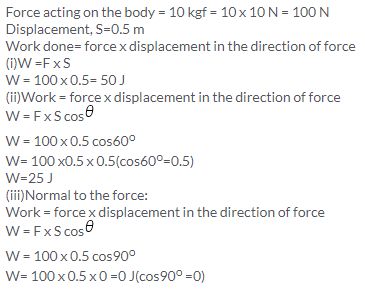Solution 2.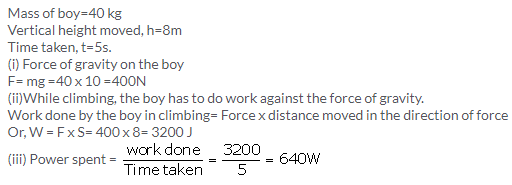Solution 3.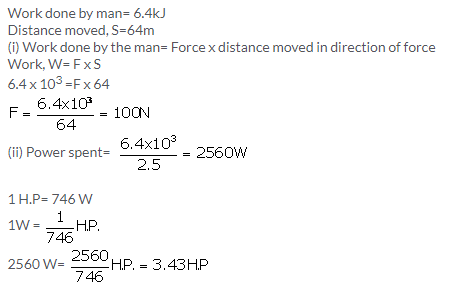Solution 4.Solution 5.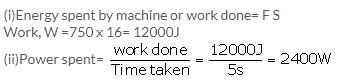Solution 6.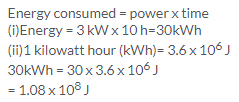Solution 7.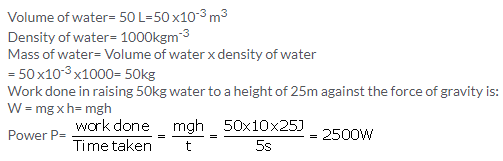Solution 8.Solution 9.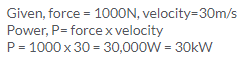Solution 10.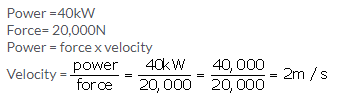Solution 12.Solution 13.

(i) The work done by persons A and B is independent of time. Hence both A and B will do the same amount of work. Hence,(ii) Power developed by the person A and B is calculated as follows:
A takes 20 s to climb the stairs while B takes 15 s, to do the same. Hence B does work at a much faster rate than A; more power is spent by B.Solution 15.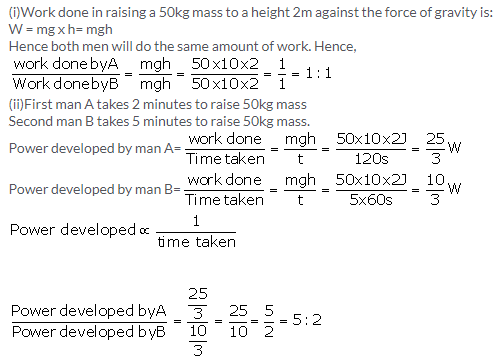Exercise 2(B)

Solution 1.

Two forms of mechanical energy are:

Solution 2.

Elastic potential energy is possessed by wound up watch spring.

Solution 3.

(a) Kinetic energy (K)
(b) Potential energy (U)
(c) Kinetic energy (K)
(d) Potential energy (U)
(e) Kinetic energy (K)
(f) Potential energy (U)

Solution 4.

Potential energy: The energy possessed by a body by virtue of its specific position (or changed configuration) is called the potential energy.
Different forms of P.E. are as listed below:

1. Gravitational potential energy: The potential energy possessed by a body due to its position relative to the centre of Earth is called its gravitational potential energy.
Example: A stone at a height has gravitational potential energy due to its raised height.
2. Elastic potential energy: The potential energy possessed by a body in the deformed state due to change in its configuration is called its elastic potential energy.
Example: A compressed spring has elastic potential energy due to its compressed state.

Solution 5.

Potential energy is possessed by the body even when it is not in motion. For example: a stone at a height has the gravitational potential energy due to its raised position.

Solution 6.

Gravitational potential energy is the potential energy possessed by a body due to its position relative to the centre of earth.
For a body placed at a height above the ground, the gravitational potential energy is measured by the amount of work done in lifting it up to that height against the force of gravity.
Let a body of mass m be lifted from the ground to a vertical height h. The least upward force F required to lift the body (without acceleration) must be equal to the force of gravity (=mg) on the body acting vertically downwards. The work done W on the body in lifting it to a height h is
W= force of gravity (mg) x displacement (h)
= mgh
This work is stored in the body when it is at a height h in the form of its gravitational potential energy.
Gravitational potential energy U= mgh

Solution 7.

The work done W on the body in lifting it to a height h is
W= force of gravity (mg) x displacement (h)
=mgh
This work is stored in the body when it is at a height h in the form of its gravitational potential energy.
Gravitational potential energy U= mgh

Solution 8.

A body in motion is said to possess the kinetic energy. The energy possessed by a body by virtue of its state of motion is called the kinetic energy.

Solution 9.Solution 10.

According to the work-energy theorem, the work done by a force on a moving body is equal to the increase in its kinetic energy.

Solution 11.

Body of mass m is moving with a uniform velocity u. A force is applied on the body due to which its velocity changes from u to v and produces an acceleration a in moving a distance S.Then,
Work done by the force= force x displacement
W = F x S———(i)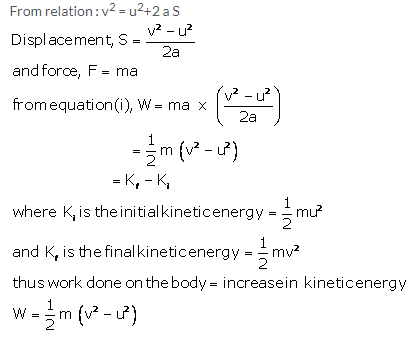Solution 12.Both the masses have same momentum p. The kinetic energy, K is inversely proportional to mass of the body.
Hence light mass body has more kinetic energy because smaller the mass, larger is the kinetic energy.

Solution 13.

Kinetic energy is related to momentum and mass as
p = √2mK
As the kinetic energy of both bodies are same, momentum is directly proportional to square root of mass.
Now, mass of body B is greater than that of body A.
Hence, body B will have more momentum than body A.

Solution 15.
The three forms of kinetic energy are:

1. Translational kinetic energy- example: a freely falling body
2. Rotational kinetic energy-example: A spinning top.
3. Vibrational kinetic energy-example: atoms in a solid vibrating about their mean position.

Solution 16.

 Potential energy (U) Kinetic energy (K) 1. The energy possessed by a body by virtue of its specific position or changed configuration is called potential energy. 1.The energy possessed by a body by virtue of its state of motion is called the kinetic energy. 2.Two forms of potential energy are gravitational potential energy and elastic potential energy. 2. Forms of kinetic energy are translational, rotational and vibrational kinetic energy. 3.Example: A wound up watch spring has potential energy. 3. For example: a moving car has kinetic energy.

Solution 17.

(a) Motion.
(b) Position.

Solution 18.

When the string of a bow is pulled, some work is done which is stored in the deformed state of the bow in the form of its elastic potential energy. On releasing the string to shoot an arrow, the potential energy of the bow changes into the kinetic energy of the arrow which makes it move.

Solution 19.

The compressed spring has elastic potential energy due to its compressed state. When it is released, the potential energy of the spring changes into kinetic energy which does work on the ball if placed on it and changes into kinetic energy of the ball due to which it flies away.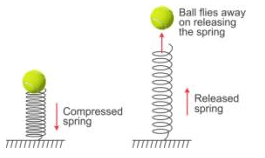Solution 20.

When water falls from a height, the potential energy stored in water at a height changes into the kinetic energy of water during the fall. On striking the ground, a part of the kinetic energy of water changes into the heat energy due to which the temperature of water rises.

Solution 21.

Yes, when force is normal to displacement, no transfer of energy takes place.

Solution 22.

Kinetic energy.

Solution 23.

The six different forms of energy are:

1. Solar energy
2. Heat energy
3. Light energy
4. Chemical or fuel energy
5. Hydro energy
6. Nuclear energy

Solution 24.

(a) Potential energy of wound up spring converts into kinetic energy.
(b) Chemical energy of petrol or diesel converts into mechanical energy (kinetic energy)
(c) Kinetic energy to potential energy
(d) Light energy changes into chemical energy
(e) Electrical energy changes into chemical energy
(f) Chemical energy changes into heat energy
(g) Chemical energy changes into heat and light energy
(h) Chemical energy changes into heat, light and sound energy

Solution 25.

(a) Electrical energy into sound energy
(b) Heat energy into mechanical energy
(c) Sound energy into electrical energy
(d) Electrical energy to mechanical energy
(e) Electrical energy into light energy
(f) Chemical energy to heat energy
(g) Light energy into electrical energy
(h) Chemical energy into heat energy
(i) Chemical energy into electrical energy
(j) Chemical energy to mechanical energy
(k) Electrical energy into heat energy
(l) Light energy into electrical energy
(m) Electrical energy into magnetic energy.

Solution 27.

No. This is because, whenever there is conversion of energy from one form to another apart of the energy is dissipated in the form of heat which is lost to surroundings.

Solution 1 (MCQ).

Potential energy
Hint: P.E. is the energy possessed by a body by virtue of its position.

Solution 2 (MCQ).

Chemical to electrical
Hint: When current is drawn from an electric cell, the chemical energy stored in it changes into electrical energy.

Numericals

Solution 1.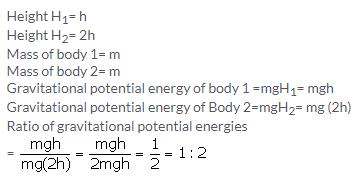Solution 2.

Mass , m=1kg
Height, h=5m
Gravitational potential energy = mgh
=1 x 10 x 5=50J

Solution 3.

Gravitational potential energy=14700 J
Force of gravity = mg= 150 x 9.8N/kg= 1470N
Gravitational potential energy= mgh
14700 =1470 x h
h=10m

Solution 4.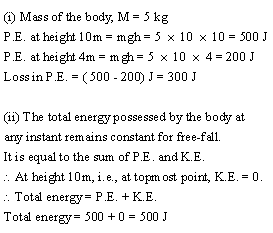Solution 5.

Mass =0.5 kg
Energy= 1 J
Gravitational potential energy= mgh
1=0.5 x10 x h
1=5h
Height, h= 0.2 m

Solution 6.

Force of gravity on boy=mg= 25 x 10 =250N
Increase in gravitational potential energy= Mg (h2-h1)
= 250 x (9-3)
=250 x6=1500 J

Solution 7.

Mass of water, m= 50kg
Height, h=15m
Gravitational potential energy= mgh
=50 x10 x 15
=7500 J

Solution 8.

Mass of man=50kg

(i) Work done by man =mgh2
=50 x 9.8 x10= 4900J

(ii) increase in his potential energy:
Height, h2= 10m
Reference point is ground, h1=0m
Gravitational potential energy= Mg (h2-h1)
= 50 x9.8x (10-0)
= 50 x 9.8 x10= 4900J

Solution 9.

F=150N

(a) Work done by the force in moving the block 5m along the slope =Force x displacement in the direction of force
=150 x 5=750 J.

(b) The potential energy gained by the block U =mgh where h =3m
=200 x 3=600 J
he potential energy gained by the block

(c) The difference i.e., 150 J energy is used in doing work against friction between the block and the slope, which will appear as heat energy.

Solution 10.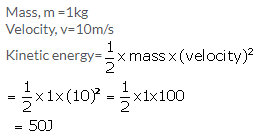Solution 11.

If the speed is halved (keeping the mass same), the kinetic energy decreases, it becomes one-fourth (since kinetic energy is proportional to the square of velocity).

Solution 13.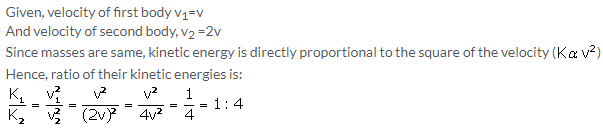Solution 14.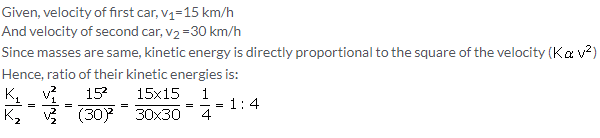Solution 15.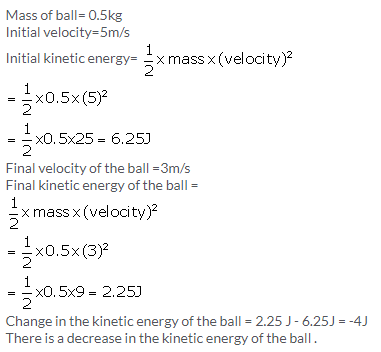Solution 16.Solution 17.Solution 18.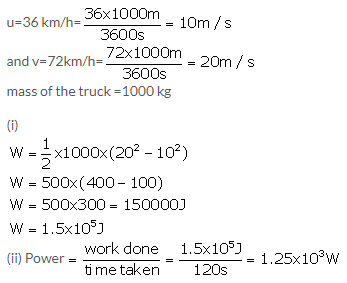Solution 19.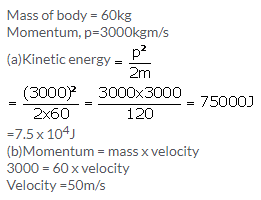Solution 20.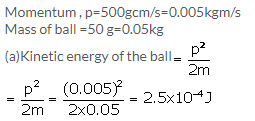Solution 21.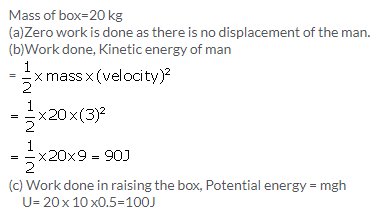Solution 22.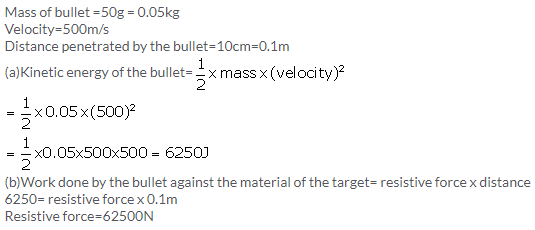Solution 23.Exercise 2(C)

Solution 1.

According to the law of conservation of energy, energy can neither be created nor can it be destroyed. It only changes from one form to another.

Solution 2.

According to the law of conservation of mechanical energy, whenever there is an interchange between the potential energy and kinetic energy, the total mechanical energy (i.e., the sum of kinetic energy K and potential energy U) remains constant i.e., K + U = constant when there are no frictional forces.

Mechanical energy is conserved only when there are no frictional forces for a given system (i.e. between body and air). Thus, conservation of mechanical energy is strictly valid only in vacuum, where friction due to air is absent.

Solution 3.

Motion of a simple pendulum and motion of a freely falling body.

Solution 4.

Kinetic energy of the body changes to potential energy when it is thrown vertically upwards and its velocity becomes zero.

Solution 5.

(a) Potential energy
(b) Potential energy and kinetic energy
(c) Kinetic energy

Solution 6.

Let a body of mass m be falling freely under gravity from a height h above the ground (i.e., from position A). Let us now calculate the sum of kinetic energy K and potential energy U at various positions, say at A (at height h above the ground), at B (when it has fallen through a distance x) and at C (on the ground).(i) At the position A (at height h above the ground):
Initial velocity of body= 0 (since body is at rest at A)
Hence, kinetic energy K =0
Potential energy U = mgh
Hence total energy = K + U= 0 + mgh =mgh—–(i)

(ii) At the position B (when it has fallen a distance x):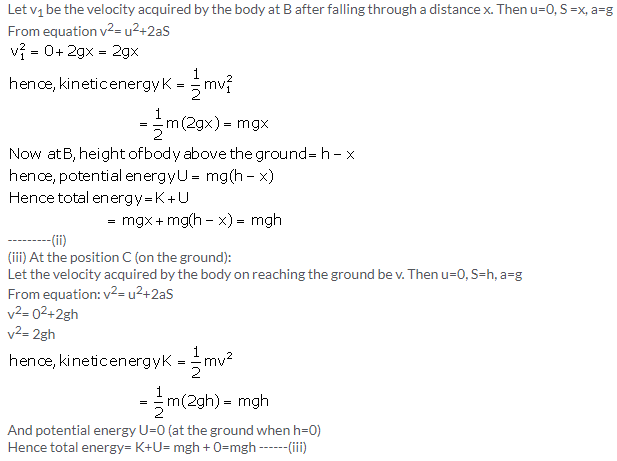Thus from equation (i), (ii) and (iii), we note that the total mechanical energy i.e., the sum of kinetic energy and potential energy always remain constant at each point of motion and is equal to initial potential energy at height h.

Solution 7.

When the bob swings from A to B, the kinetic energy decreases and the potential energy becomes maximum at B where it is momentarily at rest.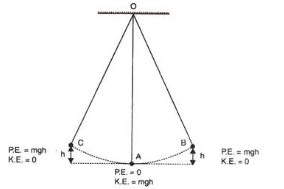From B to A, the potential energy again changes into the kinetic energy and the process gets repeated again and again.
Thus while swinging, the bob has only the potential energy at the extreme position B or C and only the kinetic energy at the resting position A. At an intermediate position (between A and B or between A and C), the bob has both the kinetic energy and potential energy, and the sum of both the energies (i.e., the total mechanical energy) remains constant throughout the swing.

Solution 8.

(a) At position A, pendulum has maximum kinetic energy and its potential energy is zero at its resting position. Hence, K=mgh and U= 0.
(b) At B, kinetic energy decreases and potential energy increases. Hence, K= 0 and U=mgh
(c) At C also, kinetic energy K= 0 and potential energy U=mgh.

Solution 9.

a) Extreme position: Potential energy
b) Mean position: Kinetic energy
c) Between mean and extreme: Both kinetic energy and potential energy

Solution 10.

The gradual decrease of useful energy due to friction etc. is called the degradation of energy.
Examples:

1. When we cook food over a fire, the major part of heat energy from the fuel is radiated out in the atmosphere. This radiated energy is of no use to us.
2. When electrical appliances are run by electricity, the major part of electrical energy is wasted in the form of heat energy.

Solution 1 (MCQ).

Potential energy of the ball at the highest point is mgh.
Hint: At the highest point, the ball momentarily comes to rest and thus its kinetic energy becomes zero.

Solution 2 (MCQ).

The sum of its kinetic and potential energy remains constant throughout the motion.
Hint: In accordance with law of conservation of mechanical energy, whenever there is an interchange between the potential energy and kinetic energy, the total mechanical energy remains constant.

Numericals

Solution 1.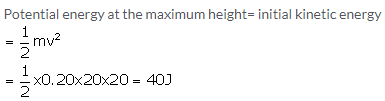Solution 2.Solution 3.

(a)Potential energy of the ball =mgh
=2 x 10 x 5=100J
(b)Kinetic energy of the ball just before hitting the ground = Initial potential energy= mgh=2x10x5=100J
(c)Mechanical energy converts into heat and sound energy.

Solution 4.Solution 5.Solution 6.More Resources for Selina Concise Class 10 ICSE Solutions